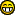News: Visit the official fractalforums.com Youtube Channel## The All New FractalForums is now in Public Beta Testing! Visit FractalForums.org and check it out!

 Pages:    Go DownAuthor Topic: 3D Fractals from the Symmetric Icons Formulas  (Read 2774 times) Description: Using the symmetric icons formulas to produce 3d fractals 0 Members and 1 Guest are viewing this topic.
Aexion
ConquerorPosts: 116The Fractal Hermit« on: October 20, 2015, 01:32:59 AM »

Hello,

I have been experimenting in creating 3D fractals from the Symmetric Icon formula.
This formula is often used to create beautiful symmetric strange attractors and in some cases, it also
is used to create 3D fractals (in Chaoscope, for example) from the attractor orbits.

Here the approach is different.
Instead of making an orbital plot, I used the escape-time algorithm (in the same way as the julia sets).
They produce 2D symmetrical sets, so I ported the formula to the spherical coordinate system, in the
same way that the Mandelbulb works. Here are some examples:The formula is here, in C code, it returns a value between 0 and 1, in the same way as the distance estimator.
It is a crude approach (I build a gradient from the level sets and the actual escape radius), but it works well for
this and for many other escape time formulas.
The formula isn't optimized, just to make it clear.

Code:
double Icon(double x, double z, double y){
double rde;
const int Maxiter=8;
double p2,p3,p4,p5,p6;
int op;

op=4;// Four Sides
double Alpha=-1.327888;
double Beta=-1.116979;
double Gamma=1.364337;
double Lambda=1.722694;

int op1=op-2;
int op2=op-1;
double p3z;
int Counter=0;
double xr,yr,zr,nx,ny,nz;
do{
xr=x;
yr=y;
zr=z;
const double p1rad=sqrt(x*x+y*y+z*z); //the standard symmetric formula doesn't use the square root, so you can remove it too
const double angle=atan2(y,x)*op2;
const double angle1=atan2(sqrt(x*x+y*y),z)*op2;
p2=cos(angle)*sinp;
p3=sin(angle)*sinp;
p5=x*p2-y*p3-z*p3z;
x=p6*x+Gamma*p2;
y=p6*y-Gamma*p3;
z=p6*z-Gamma*p3z;
Counter++;
}while(Counter<Maxiter);
const double minr=sqrt(xr*xr+yr*yr+zr*zr);
double itential=(double(Maxiter-Counter)/double(Maxiter));
double itential1=(double(Maxiter-Counter+1)/double(Maxiter));
const double totalpot=(potential*0.5+potential1*0.5);
double potiter=(itential1*totalpot+itential*(1-totalpot));
rde=pow(potiter,Counter);
return rde;
}

Oh well, hope that you like it.

Thanks

Aexion

 « Last Edit: October 20, 2015, 01:45:05 AM by Aexion »LoggedFractals all the way.. Incendia for 3D Fractals Aural for Musical Fractals
DarkBeam
Global Moderator
Fractal SeniorPosts: 2512Fragments of the fractal -like the tip of it« Reply #1 on: October 20, 2015, 08:43:40 AM »

This idea is amazingThe only downside is that it would be superslow (atan sin cos...) - Will try to do some simplification but a must try! TyLogged

No sweat, guardian of wisdom!
mclarekin
Fractal SeniorPosts: 1739« Reply #2 on: October 20, 2015, 10:01:57 AM »

@ aexion  Cool images, more formulas to add to my ever expanding "to do"  list.Logged
Aexion
ConquerorPosts: 116The Fractal Hermit« Reply #3 on: March 14, 2017, 04:40:53 AM »

Hello,

Here is a Fragmentarium shader for these fractal types.
The shader has three variations for the 3D Symmetric Icon formula and for every variation has an Abs() option.
Sadly the shader only use the Brute force rendering method. Does anyone knows any DE method that can be used here?

The formulas are unoptimized in order to keep them clear.
For how they look, just check the various examples that I have included.

The shapes are very interesting, but as I said before, right now, there's no DEAexion

ps. Since they are Symmetric Icons, I suspect that there's an strange attractor inside, but I didn't have visualized it.
pps. The Default example is uninteresting because I use it as an starting point.
ppps. They are Julia sets that's knows no Mandel due to the fact that you can't start the orbit from xyz=(0,0,0).
pppps. The controls are in the Icons section.Icons.frag (14.82 KB - downloaded 386 times.)LoggedFractals all the way.. Incendia for 3D Fractals Aural for Musical Fractals
 Pages:    Go Down
 Related Topics Subject Started by Replies Views Last postSymmetric mandelbrots as templates Theory msltoe 13 4110April 17, 2010, 03:14:17 AM by reesej2has anybody tried using ifs formulas with the mandelbuld/mandelbox formulas? 3D Fractal Generation cKleinhuis 4 6131May 05, 2010, 03:17:07 PM by Power 8Simple voxel renderer to try new formulas for 3d fractals Programming no_gravity 4 2952September 16, 2011, 10:20:14 AM by Syntopiacall for icons ... the lemmings Fractal Forums News cKleinhuis 3 3960June 14, 2012, 09:44:44 PM by cKleinhuisSymmetric group of evolution timelines Images Showcase (Rate My Fractal) Dinkydau 0 398March 25, 2014, 09:49:47 PM by Dinkydau